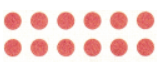# McGraw Hill Math Grade 3 Chapter 6 Lesson 1 Answer Key Multiplying by 6

## McGraw-Hill Math Grade 3 Answer Key Chapter 6 Lesson 1 Multiplying by 6

Solve

Multiply. Use drawings or objects if you need help.

Question 1.
9 × 6 = 54

Question 2.
1 × 6 = ___Explanation:
I drew circles to show the product
The product of any number multiplied with 1 is the number itself
So, 1 x 6 = 6.

Question 3.
3 × 6 = ___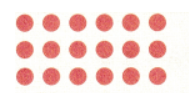Explanation:
I drew to show the product
The product of 3 and 6 is 18
So, 3 x 6 = 18.

Question 4.
5 × 6 = ____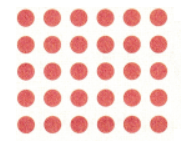Explanation:
I drew to show the product
The product of 5 and 6 is 18
So, 5 x 6 = 30.

Question 5.Explanation:
I wrote to show the product
The product of any number with 0 is 0
So, 0 x 6 = 0.

Question 6.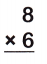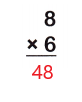Explanation:
I wrote to show the product
The product of 8 and 6 is 48
So, 8 x 6 = 48.

Question 7.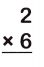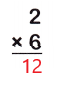Explanation:
I wrote to show the product
The product of 2 and 6 is 12
So, 2 x 6 = 12.

Question 8.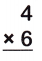Explanation:
I wrote to show the product
The product of 4 and 6 is 24
So, 4 x 6 = 24.

Question 9.
Baby giraffes are 6 feet tall. A zookeeper wants to measure 7 baby giraffes using a different piece of string each time. How many feet of string does she use?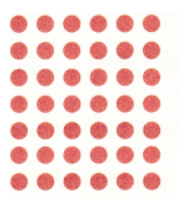Explanation:
Baby giraffes are 6 feet tall
A zookeeper wants to measure 7 baby giraffes using a different piece of string each time
Multiply 7 with 6
I drew to show the product
The product of 7 and 6 is 42
7 x 6 = 42
So, the zookeeper uses a string of 42 feet.

Question 10.
Emily plans to bring apples to share with her friends at school. She wants to give each of 6 friends 3 apples each. How many apples will Emily bring to school?Explanation:
Emily plans to bring apples to share with her friends at school
She wants to give each of 6 friends 3 apples each
Multiply 3 with 6
I drew to show the product
The product of 3 and 6 is 18
3 x 6 = 18
So, Emily bring 18 apples to school.

Question 11.
Gabe earns money by helping his neighbors shovel snow. If he can shovel 2 sidewalks in an hour, how many sidewalks can he shovel working 6 hours in a day?